• Liner Regression线性回归 线性回归是最典型的回归问题, 其目标值与所有的特征之间存在线性关系.  一元线性回归:   import matplotlib.pyplot as plt import numpy as np from sklearn.linear_model import ...
 Liner Regression线性回归
线性回归是最典型的回归问题, 其目标值与所有的特征之间存在线性关系.
一元线性回归:

import matplotlib.pyplot as plt
import numpy as np
from sklearn.linear_model import LinearRegression

rng = np.random.RandomState(1)   # 设置随机局部种子
x = 100 * rng.rand(50, 1)   # rand是[0,1] 均匀分布
y = 1.25 * x - 20 + 5*rng.randn(50, 1)   # randn是标准正态分布

model = LinearRegression(fit_intercept=True)
model.fit(x, y)  # x,y的每一行是一个样本，即要求是列向量

x_fit = np.linspace(0, 100, 1000)
y_fit = model.predict(x_fit[:, np.newaxis])
print("Model slope: ", model.coef_)
print("Model intercept:", model.intercept_)
print('方程的判定系数(R^2): %.2f' % model.score(x, y))
plt.figure(figsize=(16,12))
plt.scatter(x, y,s=10, c='k', marker='.')
plt.plot(x_fit, y_fit)
ax = plt.gca()
ax.set_aspect("equal")    # 纵横坐标单位相同
plt.grid(True)
plt.xlabel('x')
plt.ylabel('y')
plt.show()

多元线性回归(此例子为三元):
import numpy as np
from sklearn.linear_model import LinearRegression

rng = np.random.RandomState(10)   # 设置随机局部种子
x = 100 * rng.rand(50, 3)     #设置一个50行 3列  所有值乘100的随机矩阵
x1=x[:,0]
x1.shape=50,1
x2=x[:,1]
x2.shape=50,1
x3=x[:,2]
x3.shape=50,1
y = 1.25*x1+2.5*x2+3*x3+10+rng.randn(50,1)#randn是标准正态分布

model = LinearRegression(fit_intercept=True)
model.fit(x, y)  # x,y的每一行是一个样本，即要求是列向量
a = np.linspace(0,50,1000)    #从0到50创建1000个等差数列
x1_fit= a[:,np.newaxis]       #将a转置成列
x2_fit =a[:,np.newaxis]
x3_fit =a[:,np.newaxis]
x_fit = np.hstack((x1_fit,x2_fit,x3_fit))    #将x1，x2，x3合并一起
y_fit = model.predict(x_fit)      #根据x对y的预测
print("Model slope: ", model.coef_)
print("Model intercept:", model.intercept_)
print('方程的判定系数(R^2): %.2f' % model.score(x, y))
运行结果: 可以明显的看出来模型运算结果的截距和自己设置的截距是基本一样的, 判定系数无限接近于1, 那就说明这个模型是好的. 
展开全文• 多元线性回归 一个因变量依赖两个或两个以上自变量变化而变化的回归模型称为多元线性回归 ● 一元线性回归： y(x)=theta0 + theta1* x 1 ● 多元线性回归： y(x)=theta0 + theta1* x 1 + theta2* x 2 + theta3* x...
多元线性回归
一个因变量依赖两个或两个以上自变量变化而变化的回归模型称为多元线性回归

● 一元线性回归：        y(x)=theta0 + theta1* x 1
● 多元线性回归：        y(x)=theta0 + theta1* x 1 + theta2* x 2 + theta3* x 3
● 多项式（曲线）回归：    y(x)=theta0 + theta1* x 1 + theta2* (x2^2) + theta3* (x3^3) 属于多元线性回归

多元线性回归还有很多形式，比如将因变量y和自变量x取对数，取平方根，取指数等，均可看作线性回归，线性主要指参数线性

1.数据导入
import pandas as pd
import numpy as np
import matplotlib.pyplot as plt
from pandas import DataFrame,Series
from sklearn.cross_validation import train_test_split
from sklearn.linear_model import LinearRegression     #首先导入各种数据分析模块

#读取文件
examDf = DataFrame(data)


hightweightfhightmhight01.5653.01.521.5511.5753.61.541.5621.5854.21.561.5731.5954.81.581.5841.6055.41.601.5951.6156.01.621.60
2.数据绘图与检验
#绘制散点图,examDf.jt为X轴，examDf.hk为Y轴
plt.scatter(examDf.hight,examDf.weight,color = 'darkgreen',label = "Exam Data")
#添加图的标签（x轴，y轴）
plt.xlabel("hight")#设置X轴标签
plt.ylabel("weight")#设置Y轴标签
plt.show()#显示图像,一般而言纵坐标表示因变量y，这里可以调换xy顺序再试一次rDf = examDf.corr()#查看数据间的相关系数
print(rDf)

           hight    weight    fhight    mhight
hight   1.000000  0.986169  0.944395  0.792313
weight  0.986169  1.000000  0.937681  0.774636
fhight  0.944395  0.937681  1.000000  0.684220
mhight  0.792313  0.774636  0.684220  1.000000

print(examDf.describe())#数据描述，会显示最值，平均数等信息，可以简单判断数据中是否有异常值
print(examDf[examDf.isnull()==True].count())#检验缺失值，若输出为0，说明该列没有缺失值


           hight     weight     fhight     mhight
count  29.000000  29.000000  29.000000  29.000000
mean    1.700000  62.613793   1.674138   1.600690
std     0.085147   6.158718   0.066843   0.047804
min     1.560000  53.000000   1.520000   1.530000
25%     1.630000  57.200000   1.640000   1.560000
50%     1.700000  63.200000   1.680000   1.590000
75%     1.770000  66.600000   1.720000   1.630000
max     1.840000  74.400000   1.780000   1.700000
hight     0
weight    0
fhight    0
mhight    0
dtype: int64

#通过seaborn添加一条最佳拟合直线和95%的置信带，直观判断相关关系
import seaborn as sns
sns.pairplot(data, x_vars=['weight','fhight','mhight'], y_vars='hight', size=7, aspect=0.8, kind='reg')
plt.show()3.多元回归
import statsmodels.api as sm
from sklearn import model_selection
train,test =model_selection.train_test_split(examDf,test_size=0.1,train_size=0.9)
model = sm.formula.ols('hight~weight + fhight + mhight ',data =train).fit()
print(model.params)

Intercept    0.346361
weight       0.009889
fhight       0.272222
mhight       0.173867
dtype: float64

#对比预测值和实际值
test_X = test.drop(labels='hight',axis = 1)
pred = model.predict(exog = test_X)
print(pd.DataFrame({'prediction':pred,'real':test.hight}))

    prediction  real
11    1.665502  1.67
0     1.553736  1.56
9     1.648495  1.65

后续还可进一步计算均方误差，F检验，R2等统计量，这里暂时不一一介绍，本次任务主要检验数据特征，求解出回归方程系数，并展现出预测值与真实值。
展开全文• 多元线性回归：因变量和自变量之间是线性关系。 多元线性回归 参数向量W取值要满足使Loss最小，由 可得到 联立多个线性方程 转化成矩阵形式 求解 代码实现 import tensorflow as tf import numpy as np ...
多元回归：回归分析中包括两个或两个以上的自变量。 多元线性回归：因变量和自变量之间是线性关系。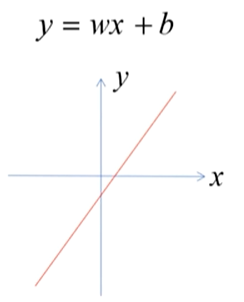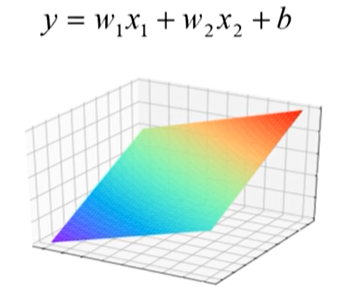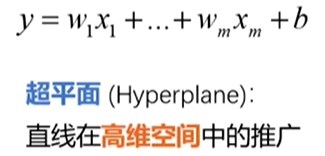多元线性回归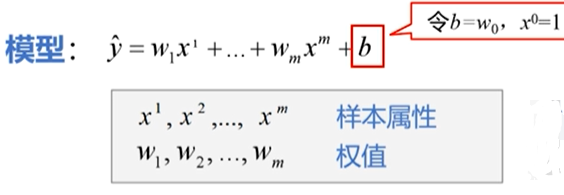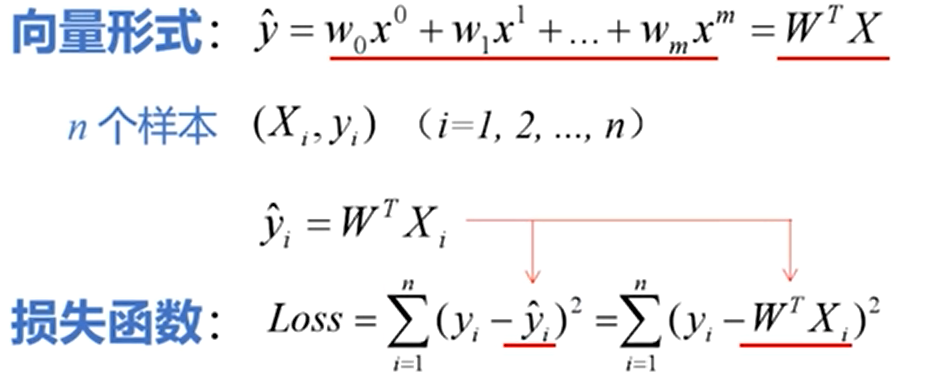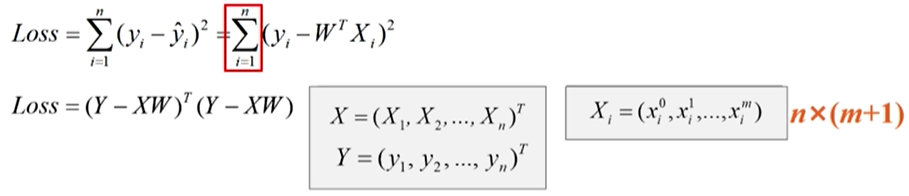参数向量W取值要满足使Loss最小，由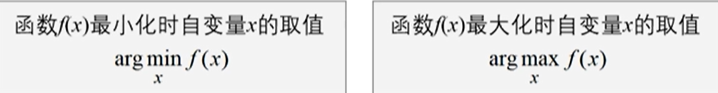可得到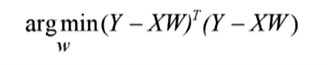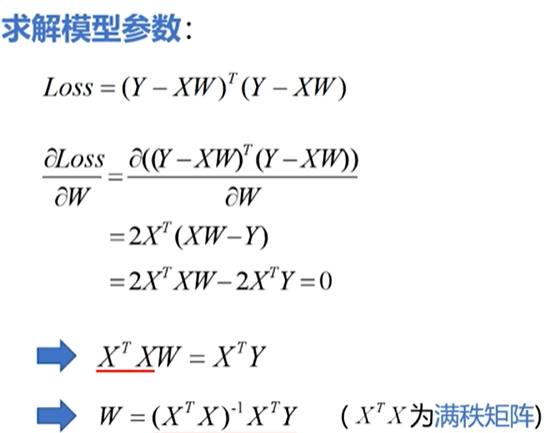联立多个线性方程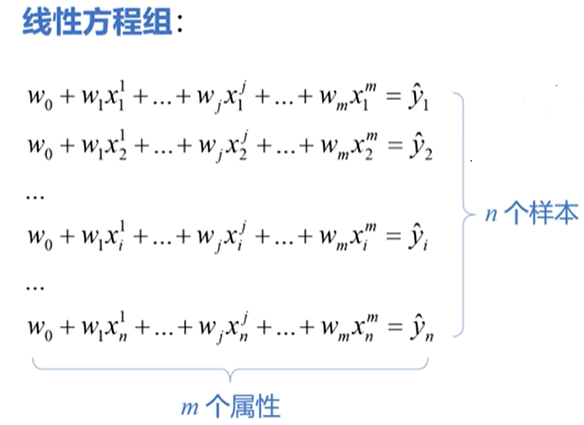转化成矩阵形式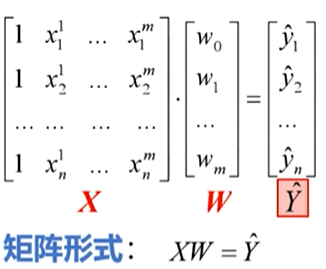求解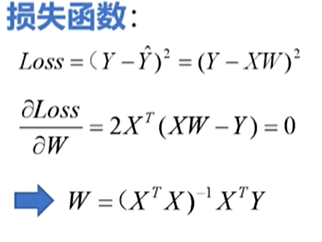代码实现
import tensorflow as tf
import numpy as np

#设置图像中显示中文
plt.rcParams['font.sans-serif'] = ['SimHei']
#房子面积
x1 = np.array([137.97,104.50,100.00,124.32,79.2,99.,124.,114.,106.69,138.05,53.75,46.91,68.,63.02,81.26,86.21])
x2 = np.array([3,2,2,3,1,2,3,2,2,3,1,1,1,1,2,2]) # 房间数
y = np.array([145.,110.,93.,116.,65.32,104.,118.,91.,62.,133.,51.,45.,78.5,69.65,75.69,95.3])# 价格
print(x1.shape,x2.shape,y.shape)
x0 = np.ones(len(x1))
X=np.stack((x0,x1,x2),axis=1)  # 把三个一维数组合并成一个二维数组
print(X)
Y = np.array(y).reshape(-1,1) #得到一个16维1列的二维数组

Xt = np.transpose(X) #计算X'
XtX_1 = np.linalg.inv(np.matmul(Xt,X)) # 计算(X'X)-1次方
XtX_1_Xt = np.matmul(XtX_1,Xt) # 计算(X'X)-1次方乘以X'
W = np.matmul(XtX_1_Xt,Y) #W=((X'X)-1次方)X'Y
W = W.reshape(-1)
print("多元线性回归方程：")
print("Y=",W,"*x1+",W,"*x2+",W)
print("请输入房屋面积和房间数，预测房屋销售价格：")
x1_test = float(input("商品房面积："))
x2_test = int(input("房间数："))

y_pred = W*x1_test+W*x2_test+W
print("预测价格:",round(y_pred,2),"万元")


可视化
import numpy as np
import matplotlib.pyplot as plt
from mpl_toolkits.mplot3d import Axes3D

#设置图像中显示中文
plt.rcParams['font.sans-serif'] = ['SimHei']

x1 = np.array([137.97,104.50,100.00,124.32,79.2,99.,124.,114.,106.69,138.05,53.75,46.91,68.,63.02,81.26,86.21])
x2 = np.array([3,2,2,3,1,2,3,2,2,3,1,1,1,1,2,2])
y = np.array([145.,110.,93.,116.,65.32,104.,118.,91.,62.,133.,51.,45.,78.5,69.65,75.69,95.3])
print(x1.shape,x2.shape,y.shape)
x0 = np.ones(len(x1))
X=np.stack((x0,x1,x2),axis=1)  # 把三个一维数组合并成一个二维数组
print(X)
Y = np.array(y).reshape(-1,1) #得到一个16维1列的二维数组

Xt = np.transpose(X) #计算X'
XtX_1 = np.linalg.inv(np.matmul(Xt,X)) # 计算(X'X)-1次方
XtX_1_Xt = np.matmul(XtX_1,Xt) # 计算(X'X)-1次方乘以X'
W = np.matmul(XtX_1_Xt,Y) #W=((X'X)-1次方)X'Y
W = W.reshape(-1)
# print("多元线性回归方程：")
# print("Y=",W,"*x1+",W,"*x2+",W)
# print("请输入房屋面积和房间数，预测房屋销售价格：")
# x1_test = float(input("商品房面积："))
# x2_test = int(input("房间数："))
#
# y_pred = W*x1_test+W*x2_test+W
# print("预测价格:",round(y_pred,2),"万元")
y_pred = W*x1+W*x2+W

fig = plt.figure(figsize=(8,6))
ax3d = Axes3D(fig) # 创建3D绘图对象
# ax3d.view_init(elev=0,azim=-90) # 改变图形视角elev表示水平高度，azim表示水平旋转角度

ax3d.scatter(x1,x2,y,color="b",marker="*")
ax3d.set_xlabel("Area",color="r",fontsize=16)
ax3d.set_ylabel("Room",color="r",fontsize=16)
ax3d.set_zlabel("Price",color="r",fontsize=16)
ax3d.set_yticks([1,2,3]) # 设置y轴的坐标轴刻度
ax3d.set_zlim3d(30,160) # 设置z轴的坐标轴范围

#绘制平面图
X1,X2 = np.meshgrid(x1,x2)
Y_PRED=W+W*X1+W*X2

fig = plt.figure()
ax3d = Axes3D(fig)

ax3d.plot_surface(X1,X2,Y_PRED,cmap="coolwarm")

ax3d.set_xlabel("Area",color="r",fontsize=14)
ax3d.set_ylabel("Room",color="r",fontsize=14)
ax3d.set_zlabel("Price",color="r",fontsize=14)
ax3d.set_yticks([1,2,3])

# 绘制散点图和线框图
plt.rcParams['font.sans-serif'] = ['SimHei']
fig = plt.figure()
ax3d = Axes3D(fig)

ax3d.scatter(x1,x2,y,color="b",marker="*",label="销售记录")
ax3d.scatter(x1,x2,y_pred,color="r",label="预测房价")
ax3d.plot_wireframe(X1,X2,Y_PRED,color="c",linewidth=0.5,label="拟合平面")

ax3d.set_xlabel("Area",color="r",fontsize=14)
ax3d.set_ylabel("Room",color="r",fontsize=14)
ax3d.set_zlabel("Price",color="r",fontsize=14)
ax3d.set_yticks([1,2,3])
plt.suptitle("商品房销售回归模型",fontsize=20)
plt.legend(loc="upper left")
plt.show()

结果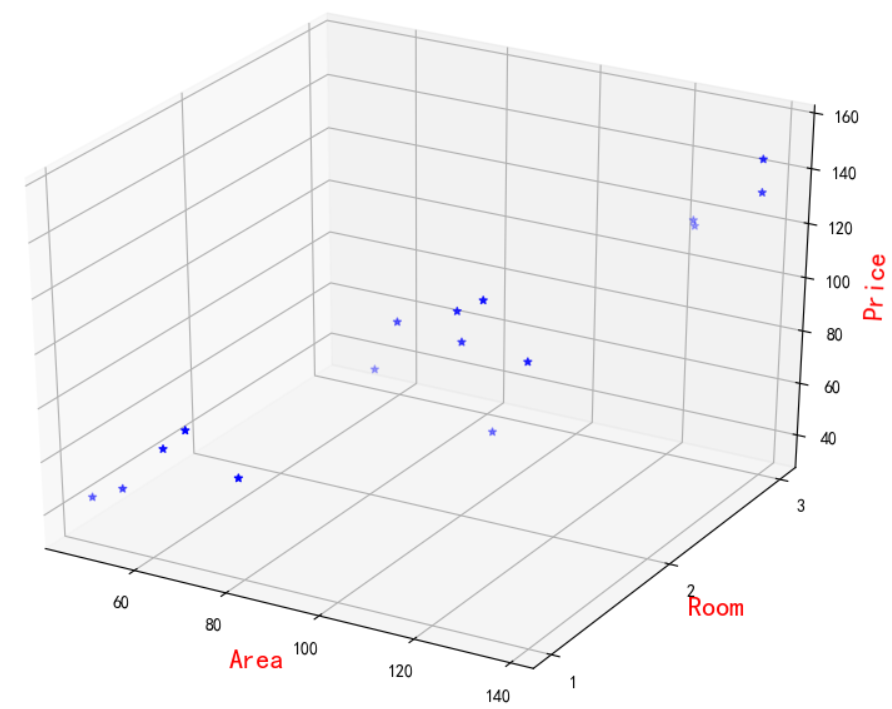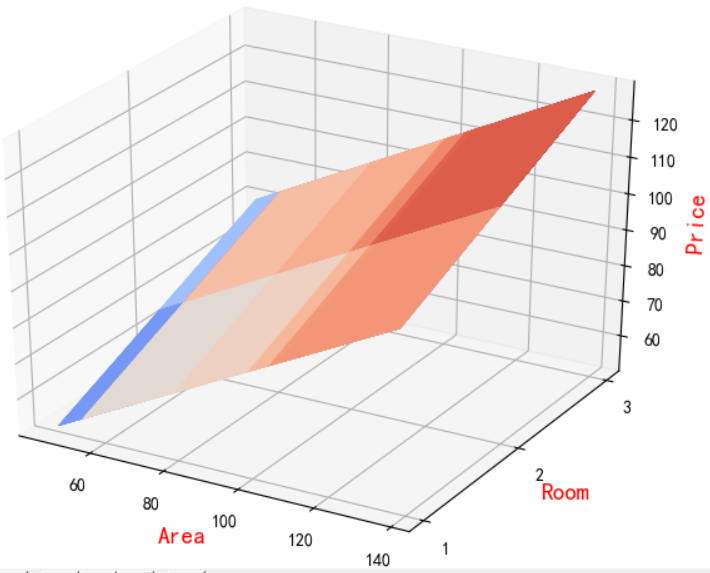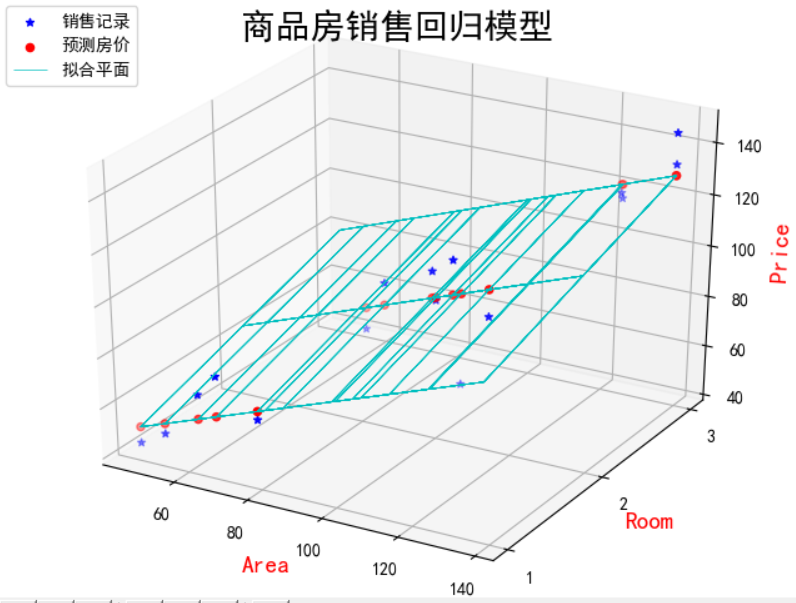展开全文机器学习 python 深度学习
• 文章目录原理以及公式【1】一元线性回归问题【2】多元线性回归问题【3】学习率【4】流程分析（一元线性回归）【5】流程分析（多元线性回归）归一化原理以及每种归一化适用的场合一元线性回归代码以及可视化结果多元...


文章目录
原理以及公式【1】一元线性回归问题【2】多元线性回归问题【3】学习率【4】流程分析（一元线性回归）【5】流程分析（多元线性回归）归一化原理以及每种归一化适用的场合

一元线性回归代码以及可视化结果多元线性回归代码以及可视化结果总结

原理以及公式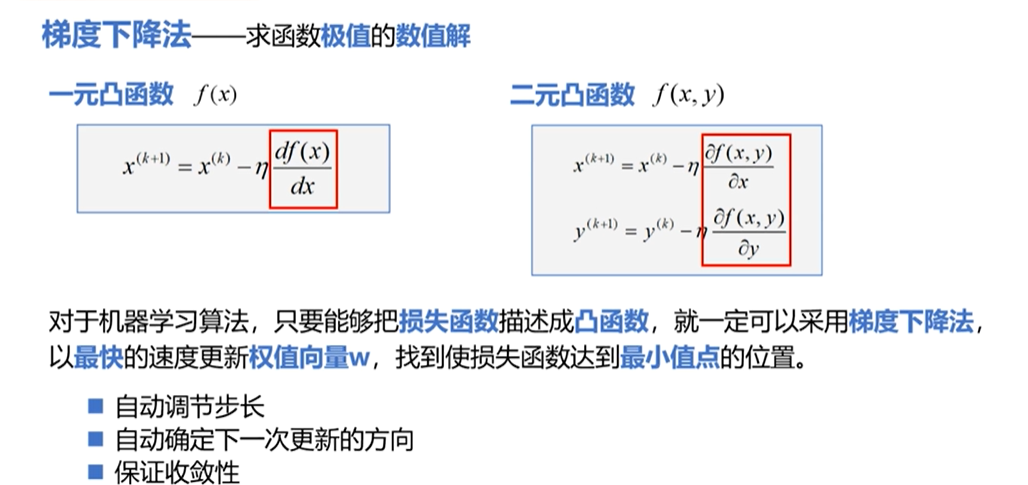【1】一元线性回归问题
原函数是一元函数(关于x)，它的损失函数是二元函数(关于w和b)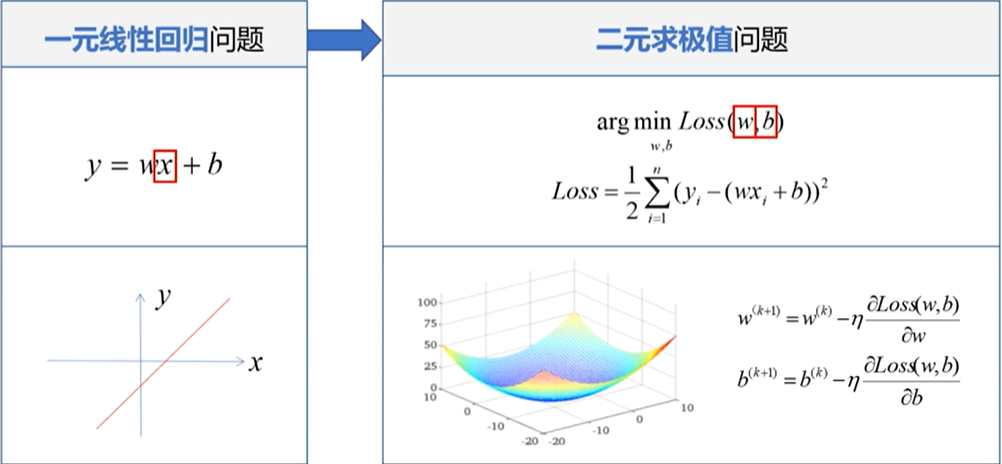这里介绍两种损失函数：平方损失函数和均方差损失函数【2】多元线性回归问题
X和W都是m+1维的向量，损失函数是高维空间中的凸函数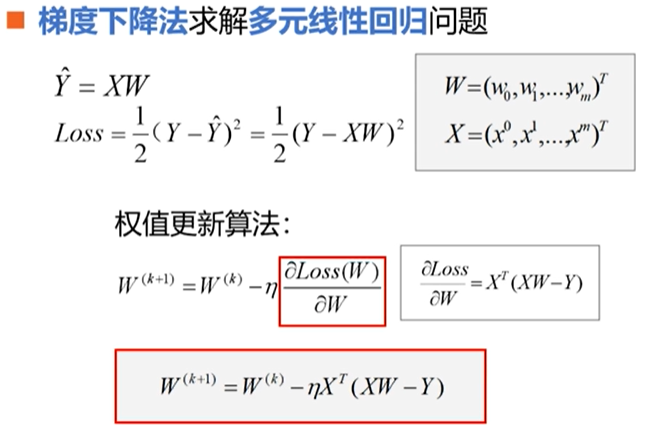【3】学习率
学习率属于超参数(超参数:在开始学习之前设置，不是通过训练得到的) 可以选择在迭代次数增加时减少学习率大小. 下图是学习率正常或较小、稍大、过大的迭代图。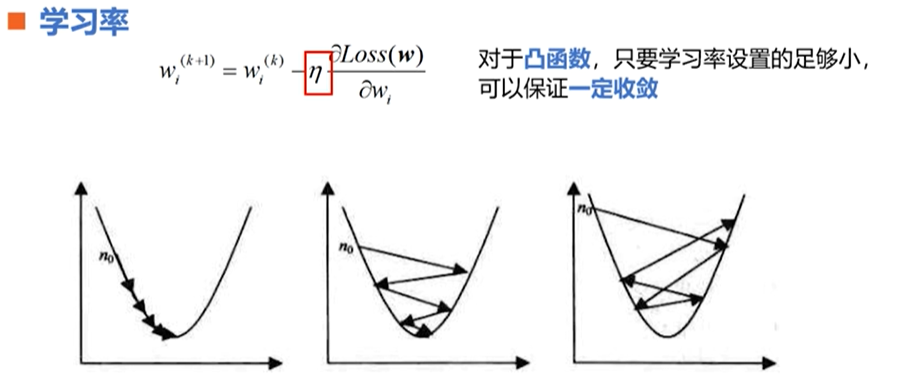【4】流程分析（一元线性回归）
过程分析：

1、加载样本数据x,y 2、设置超参数学习率，迭代次数 3、设置模型参数初值w0， b0 4、训练模型w, b 5、结果可视化

														流程图：【5】流程分析（多元线性回归）
归一化原理以及每种归一化适用的场合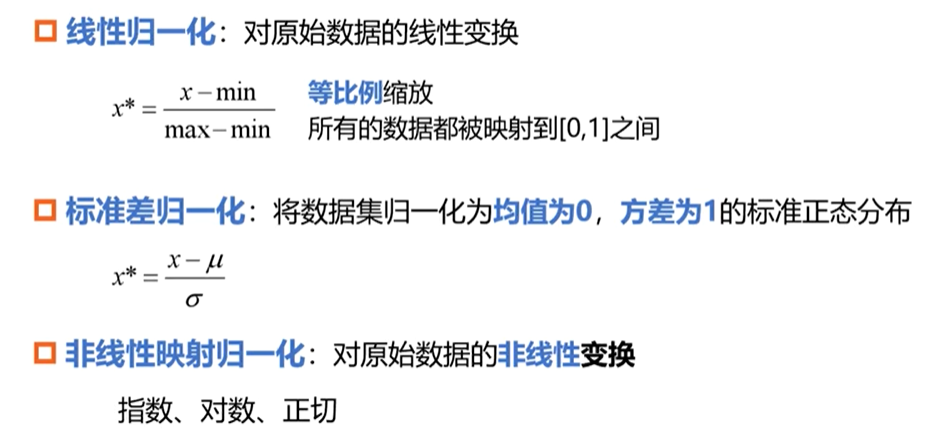线性归一化：适用于样本分布均匀且集中的情况，如果最大值(或者最小值)不稳定，和绝大数样本数据相差较大，使用这种方法得到的结果也不稳定.为了抑制这个问题，在实际问题中可以用经验值来代替最大值和最小值 标准差归一化适用于样本近似正态分布，或者最大最小值未知的情况，有时当最大最小值处于孤立点时也可以使用标准差归一化 非线性映射归一化，通常用于数据分化较大的情况（有的很大有的很小） 总结：样本属性归一化需要根据属性样本分布规律定制

过程分析：

加载样本数据area,room,price 数据处理归一化，X，Y 设置超参数学习率,迭代次数 设置模型参数初值W0(w0,w1,w2) 训练模型W 结果可视化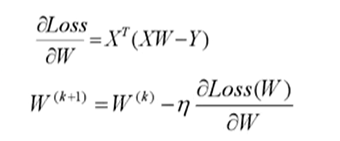一元线性回归代码以及可视化结果
#解析法实现一元线性回归
# #Realization of one variable linear regression by analytic method
#导入库
import numpy as np
import matplotlib.pyplot as plt
#设置字体
plt.rcParams['font.sans-serif'] =['SimHei']
#加载样本数据
x=np.array([137.97,104.50,100.00,124.32,79.20,99.00,124.00,114.00,106.69,138.05,53.75,46.91,68.00,63.02,81.26,86.21])
y=np.array([145.00,110.00,93.00,116.00,65.32,104.00,118.00,91.00,62.00,133.00,51.00,45.00,78.50,69.65,75.69,95.30])
#设置超参数,学习率
learn_rate=0.00001
#迭代次数
iter=100
#每10次迭代显示一下效果
display_step=10
#设置模型参数初值
np.random.seed(612)
w=np.random.randn()
b=np.random.randn()
#训练模型
#存放每次迭代的损失值
mse=[]
for i in range(0,iter+1):
#求偏导
dL_dw=np.mean(x*(w*x+b-y))
dL_db=np.mean(w*x+b-y)
#更新模型参数
w=w-learn_rate*dL_dw
b=b-learn_rate*dL_db
#得到估计值
pred=w*x+b
#计算损失(均方误差)
Loss=np.mean(np.square(y-pred))/2
mse.append(Loss)
#显示模型
#plt.plot(x,pred)
if i%display_step==0:
print("i:%i,Loss:%f,w:%f,b:%f"%(i,mse[i],w,b))
#模型和数据可视化
plt.figure(figsize=(20,4))
plt.subplot(1,3,1)
#绘制散点图
#张量和数组都可以作为散点函数的输入提供点坐标
plt.scatter(x,y,color="red",label="销售记录")
plt.scatter(x,pred,color="blue",label="梯度下降法")
plt.plot(x,pred,color="blue")

#设置坐标轴的标签文字和字号
plt.xlabel("面积（平方米）",fontsize=14)
plt.xlabel("价格（万元）",fontsize=14)

#在左上方显示图例
plt.legend(loc="upper left")

#损失变化可视化
plt.subplot(1,3,2)
plt.plot(mse)
plt.xlabel("迭代次数",fontsize=14)
plt.ylabel("损失值",fontsize=14)
#估计值与标签值比较可视化
plt.subplot(1,3,3)
plt.plot(y,color="red",marker="o",label="销售记录")
plt.plot(pred,color="blue",marker="o",label="梯度下降法")
plt.legend()
plt.xlabel("sample",fontsize=14)
plt.ylabel("price",fontsize=14)
#显示整个绘图
plt.show()多元线性回归代码以及可视化结果
#解析法实现多元线性回归
#Realization of multiple linear regression by analytic method
#导入库与模块
import numpy as np
import matplotlib.pyplot as plt
from mpl_toolkits.mplot3d import Axes3D
#=======================【1】加载样本数据===============================================
area=np.array([137.97,104.50,100.00,124.32,79.20,99.00,124.00,114.00,106.69,138.05,53.75,46.91,68.00,63.02,81.26,86.21])
room=np.array([3,2,2,3,1,2,3,2,2,3,1,1,1,1,2,2])
price=np.array([145.00,110.00,93.00,116.00,65.32,104.00,118.00,91.00,62.00,133.00,51.00,45.00,78.50,69.65,75.69,95.30])
num=len(area) #样本数量
#=======================【2】数据处理===============================================
x0=np.ones(num)
#归一化处理，这里使用线性归一化
x1=(area-area.min())/(area.max()-area.min())
x2=(room-room.min())/(room.max()-room.min())
#堆叠属性数组，构造属性矩阵
#从(16,)到(16,3),因为新出现的轴是第二个轴所以axis为1
X=np.stack((x0,x1,x2),axis=1)
print(X)
#得到形状为一列的数组
Y=price.reshape(-1,1)
print(Y)
#=======================【3】设置超参数===============================================
learn_rate=0.001
#迭代次数
iter=500
#每10次迭代显示一下效果
display_step=50
#=======================【4】设置模型参数初始值===============================================
np.random.seed(612)
W=np.random.randn(3,1)
#=======================【4】训练模型=============================================
mse=[]
for i in range(0,iter+1):
#求偏导
dL_dW=np.matmul(np.transpose(X),np.matmul(X,W)-Y)   #XT(XW-Y)
#更新模型参数
W=W-learn_rate*dL_dW
#得到估计值
PRED=np.matmul(X,W)
#计算损失(均方误差)
Loss=np.mean(np.square(Y-PRED))/2
mse.append(Loss)
#显示模型
#plt.plot(x,pred)
if i % display_step==0:
print("i:%i,Loss:%f"%(i,mse[i]))
#=======================【5】结果可视化============================================
plt.rcParams['font.sans-serif'] =['SimHei']
plt.figure(figsize=(12,4))
#损失变化可视化
plt.subplot(1,2,1)
plt.plot(mse)
plt.xlabel("迭代次数",fontsize=14)
plt.ylabel("损失值",fontsize=14)
#估计值与标签值比较可视化
plt.subplot(1,2,2)
PRED=PRED.reshape(-1)
plt.plot(price,color="red",marker="o",label="销售记录")
plt.plot(PRED,color="blue",marker="o",label="预测房价")
plt.xlabel("sample",fontsize=14)
plt.ylabel("price",fontsize=14)
plt.legend()
plt.show()总结
注意点：选择归一化方式

喜欢的话点个赞和关注呗！ 
展开全文可视化 python 深度学习 机器学习 数据分析
• 若是包含多个因变量则是多元线性回归，即y=θ0+θ1X1+θ2X2+…+θnXn。 简单来说就是给你一堆数据，你从几个不同变量中找出它们之间的函数关系，并求出这些匹配不同变量的系数，如θ0，θ1等。 梯度下降算法: 梯度...
• 多元线性回归程序示例 类似的，我们也可以实现多元线性回归。这里，我们需要创建多个特征（x），我们也可以像之前程序那样，随机生成多个特征，不过，这里，我们使用sklearn库提供的更方面的方法。 from sklearn....可视化 机器学习
• 多元线性回归：含有多个特征及多个自变量（输入） 公式： 参数： 损失函数： 梯度下降： Repeat{ (j=0,1,2,3,4,...,n) } 下面是未使用sklearn的代码 import numpy as np import matplotlib.pyplot as ...
• matlab多元线性回归及显著性分析给各位高手：小弟有一些数据需要回归分析(非线性)及显著性检验(回归模型，次要项，误差及失拟项纯误差，F值和P值)，求大侠帮助，给出程序，不胜感激。模型：DA TA=... %DA TA前三列...regress 梯度下降法
• 大家好，我是天空之城，今天给大家带来小福利，带你快速了解一元线性回归方程模拟和多元线性回归方程模拟 主要用来进行数据的相关性分析 之前在研究生阶段用过一个叫origin的数据处理软件，只要是科研数据都可以用这...数据分析
• 多元线性回归，主要是研究一个因变量与多个自变量之间的相关关系，跟一元回归原理差不多，区别在于影响因素（自变量）更多些而已，例如：一元线性回归方程 为： 毫无疑问，多元线性回归方程应该为： 上图中的 x1,...
• 今天给大家分享的科研教程是多元线性回归、指数拟合和非线性曲面拟合。 一、多元线性回归 多元线性回归用于分析多个自变量与一个因变量之间的线性关系。下式为一般多元线性方程。 Y=A+B1X1+B2X2…+BnXn Origin在...origin教程
• 机器学习入门之多元线性回归及其 Python 实现机器学习
• 多元线性回归问题——波士顿房价预测 波士顿房价预测数据集包括506个样本，每个样本包括12个特征变量和该地区的平均房价（单价）显然和多个特征变量相关，不是单变量线性回归（一元线性回归）问题，选择多个特征变量...深度学习 tensorflow python
• python 多元线性回归boston房价预测 jupyter python3环境下利用处理后的boston房价数据集建立多元线性回归模型 数据集各特征描述： 导入使用到的相关包 将使用statsmodels库的ols函数建模，variance_inflation_...
• 线性回归（又名普通最小二乘法） 线性回归，或者普通最小二乘法，是回归问题最简单也是最经典的线性方法。线性回归寻找参数w和b，使得对训练集的预测值与真实的回归目标值y之间的均方误差最小。均方误差是预测值与...机器学习
• 继上次对成都房租进行可视化后，这次简单的做一个成都房租与面积、所属区的多元回归分析。 数据集一共有3w数据，每一组观测值对应一个房租情况，其中: 价钱:一个月房租，单位人民币。 面积:租房的面积，单位平方米。...python
• 机器学习基础之多元线性回归 描述了假设函数、求解方法（梯度法和正规方程）、基于Octave/MATLAB的向量化表达方法。机器学习 Octave MATLAB
• 多元线性回归，主要是研究一个因变量与多个自变量之间的相关关系，跟一元回归原理差不多，区别在于影响因素（自变量）更多些而已，例如：一元线性回归方程 为： 毫无疑问，多元线性回归方程应该为： 上图中的 x1,...
• 1、单变量线性回归预测模型数据操作data（:,1）返回数据第一列length (a)返回对象a的长度。空对象的长度为0，标量为1，矢量元素的数量。 对于矩阵或N维对象，长度是沿最大维度的元素数量（等于max（size（a）））...机器学习
• 多元线性回归表达式 其中， X=[1,x1,⋯ ,xn] X=[1,x_1,\cdots,x_n] X=[1,x1​,⋯,xn​] W=[w0,w1,⋯ ,wn] W=[w_0,w_1,\cdots,w_n] W=[w0​,w1​,⋯,wn​] 损失函数 其中X,YX,YX,Y是矩阵。 梯度下降 令H=Y−XWTH=...矩阵求导 python 机器学习
• SPSS--回归-多元线性回归模型案例解析！(一）  多元线性回归，主要是研究一个因变量与多个自变量之间的相关关系，跟一元回归原理差不多，区别在于影响因素（自变量）更多些而已，例如：一元线性回归方程 为： ...
• 文章目录记录TensorFlow听课笔记一，机器学习基础1.1一元线性回归 一，机器学习基础 1.1一元线性回归 模型：y =wx+b 模型变量：x 模型参数 w：权重（weights） b：偏置值（bias） ...
• 解析法实现一元线性回归 代码： #加载样本数据 x=[137.97,104.50,100.00,124.32,79.20,99.00,124.00,114.00,106.69,138.05,53.75,46.91,68.00,63.02,81.26,86.21] y=[145.00,110.00,93.00,116.00,65.32,104.00,118....可视化 python 机器学习 数据分析 numpy
• 多元线性回归 yi=β0+β1*xi1+β2*xi2+……+ei； 原假设Ho为βj=0，即线性回归的系数为0.通常使用Pr>|t|小于α时拒绝原假设Ho，认为系数不为0；否则接受原假设Ho，认为系数为0，系数没有通过检验。 某水稻...statistics output input...